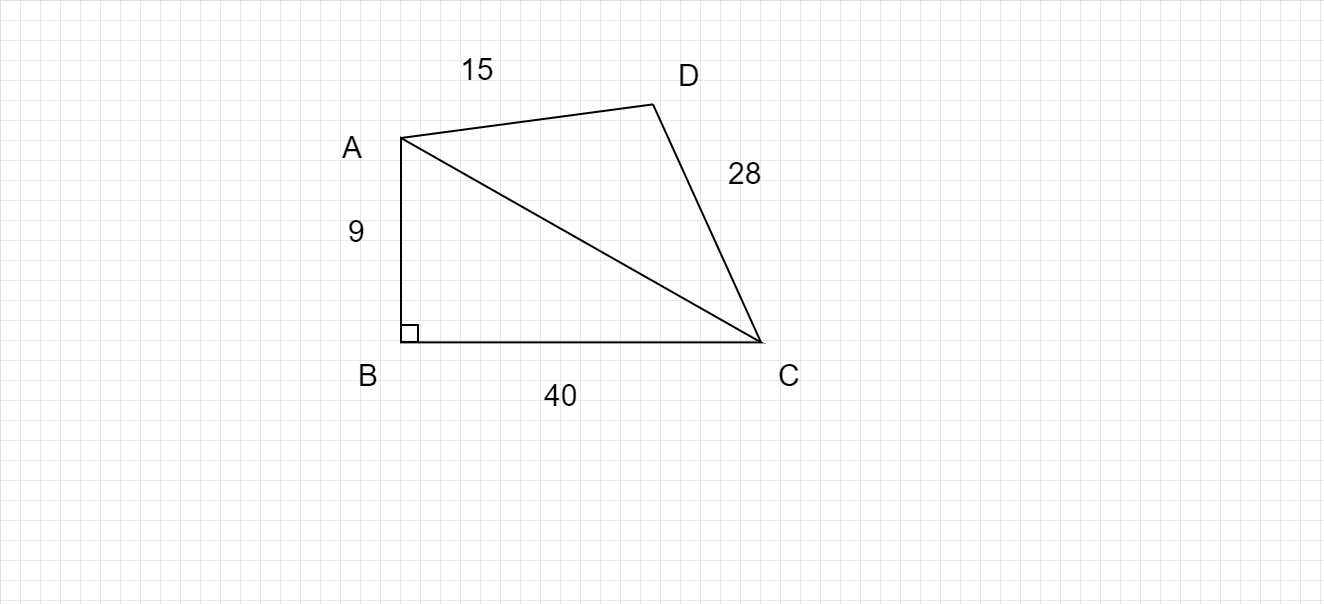# How to find the area of irregular shapes ?

Area of irregular shapes :

To find the area of an Irregular Shape,

• Divide the irregular shape in two or more regular shapes.
• Use the required formulas to calculate the area of such shapes and add them together to obtain the total area.

Example :In the above figure, the irregular quadrilateral is divided into two triangles, so that we can find the area of triangles and add them to get the area of the quadrilateral.

Updated on: 10-Oct-2022

69 Views